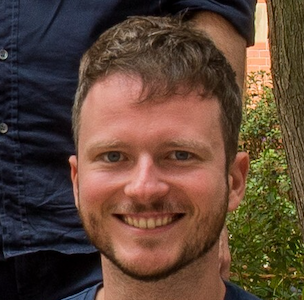# T-2: Putting perception into action

Saturday, 27 August, 10:30 - 12:15 Pacific Time (UTC -7)
Location: Grand Ballroom B-C
Tutorial

Tutorial Materials:

Abstract:

Normative computational models of behavior strive to explain why behavior unfolds the way it does. These models have been highly successful in explaining a vast array of phenomena in neuroscience, cognitive science, and related fields, e.g. ideal observer models in sensory neuroscience and optimal control models in motor control. The power of these approaches derives from the combination of controlled experimental designs with their associated computational analyses, e.g. perceptual forced-choice decisions with signal detection theory or repeated reaching movements with optimal control models. Normative models have been central to the reverse-engineering approach to understanding cognition, whereby researchers describe a task on the computational level and then derive hypotheses for possible algorithms and implementations.

To construct a normative model, one typically describes the experimental task devised by the researcher in terms of its statistical structure and the goals of a rational agent. One commonly assumes that subjects, after substantial training, know both the statistical structure of the tasks and the rewards implemented in the experiment by the researcher. Under this assumption, one uses the true generative model of the experimental stimuli and compares predictions of the normative model with observed behavior - an approach known as ideal observer analysis. However, subjects potentially may have different internal beliefs about the experiment’s statistical structure or may experience intrinsic costs for behavior such as effort, differing from the researcher’s instructions. Similarly, in naturalistic behavior, participants’ internal model of the environment may differ from the true one and subjective costs and benefits may not exactly match the rewards the researcher has envisioned. To deal with such cases, we use an inverse approach normative modeling: to infer under which internal model and reward function the observed behavior is rational.

We present previous research from our lab systematically moving from ideal observer models toward optimal actors, bounded actors, and subjective actors with possibly mistaken internal beliefs about stimuli and environmental dynamics. We then show how these models can be inverted to infer the parameters of the subject’s internal model. Particularly, we present recent work that applies this methodology to ‘continuous psychophysics’, an approach that abandons the rigid structure of classical psychophysics tasks, in which highly trained observers complete hundreds of independent trials. Instead, subjects perform sequential behavioral adjustments to dynamic stimuli, e.g. in a continuous tracking task. This approach promises to transform the field, because it requires orders of magnitude less time to collect data, can be used with untrained subjects, and has been reported to be perceived as intuitive. We introduce an analysis framework for such tasks based on inverse optimal control. The proposed method allows inferring subjective costs and benefits, which may differ from the external rewards, and subjective beliefs, which may differ from the external stimulus properties. This opens up the possibility of relating neuronal activity to these internal, cognitive quantities to better understand how the brain produces behavior in uncertain dynamic environments. Finally, because the proposed method is probabilistic, it quantifies the uncertainty the researcher should have about the estimated quantities.

Tutorial Plan:

In this tutorial, we will give a detailed look at optimal control models of continuous psychophysics tasks and our method for inverse optimal control. Specifically, participants will learn step by step how to build an optimal control agent for a target tracking task with perceptual uncertainty, action variability, behavioral costs, and subjective beliefs. This includes a brief introduction to optimal control in the linear-quadratic Gaussian (LQG) framework. We will then give guidance on how to turn an intuitive understanding of a task into concrete choices for stochastic linear dynamical systems and cost functions. To introduce inverse optimal control, we emphasize the distinction between the subject's point of view and the researcher's point of view using probabilistic graphical models. From the subject's point of view, an internal model describes the task dynamics and subjective goals. From the researcher's point of view, a statistical model of the subject's behavior is used to infer the parameters of the subject's internal model. Participants will get an intuitive understanding of the derivations for probabilistic inverse optimal control based on this conceptual framework.

The second half of the tutorial is a hands-on lesson that goes into detail about the implementation of (inverse) optimal control. We showcase our Python library for inverse optimal control in the LQG framework, which is based on the automatic differentiation package jax. In a Colab notebook, participants will learn how to use the library to define and simulate optimal control agents and develop an intuition for how the model parameters influence the trajectories generated by the agent. We will also show how the mathematical derivations for probabilistic inverse optimal control from the first part of the tutorial are implemented and how to perform Bayesian inference using the probabilistic programming package numpyro. Example experiments include a manual tracking task with humans and a gaze tracking task with non-human primates.Constantin Rothkopf